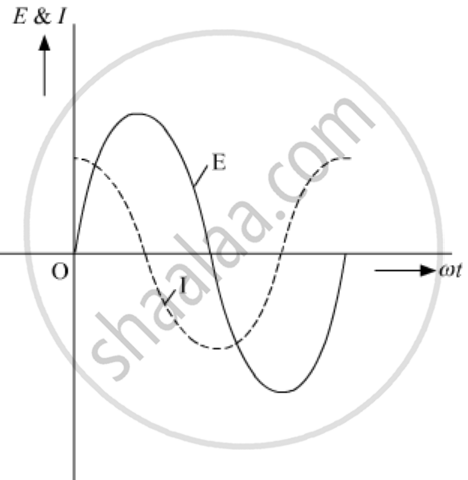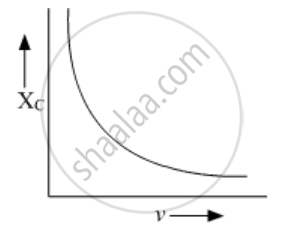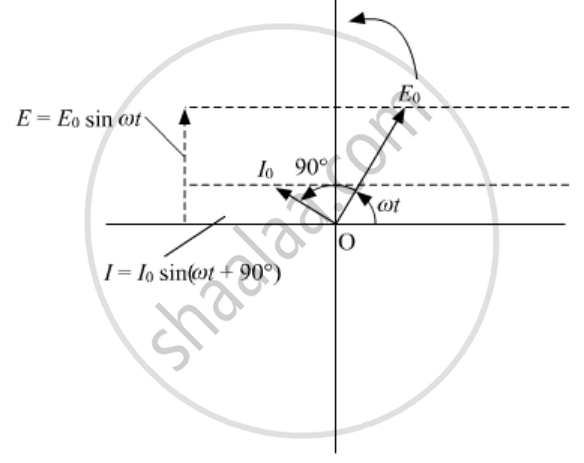Share

# A Device X is Connected Across an Ac Source of Voltage V = V0 Sin ωT. the Current Through X is Given Identify the Device X and Write the Expression for Its Reactance. and and Draw Graphs Showing the Variation of Voltage and Current with Time Over One Cycle of Ac, for X. and How Does the Reactance of the Device X Vary with the Frequency of the Ac? Show this Variation Graphically - Physics

#### Question

A device X is connected across an ac source of voltage V = V0 sin ωt. The current through X is given as

I = I_0 sin (omega t + pi/2 )

1) Identify the device X and write the expression for its reactance.

2) Draw graphs showing the variation of voltage and current with time over one cycle of ac, for X.

3) How does the reactance of the device X vary with the frequency of the ac? Show this variation graphically.

4) Draw the phasor diagram for the device X.

#### Solution

1) Since the current is leading the voltage by 90 degrees, the device X is a capacitor. The expression for reactance is

X_c = 1/(omegac) = 1/(2pivc)

2)3) X_c = 1/(omegac) = 1/(2pivc)

where v = frequency of the signal.

The reactance varies inversely with frequency.4)Is there an error in this question or solution?